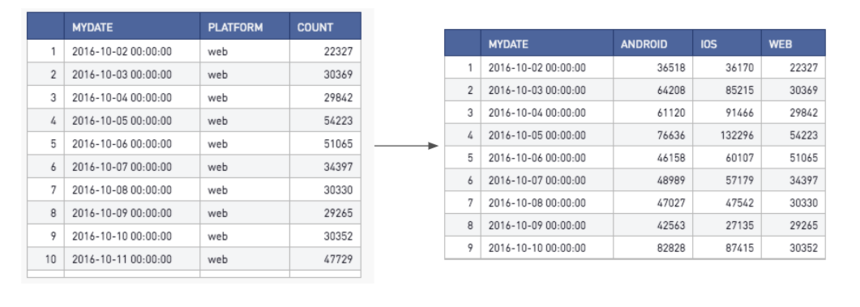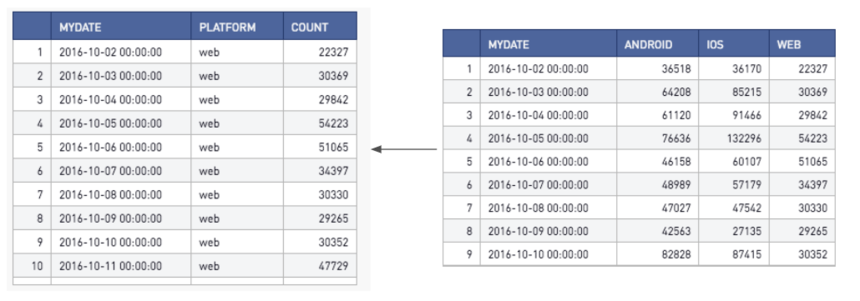Pivoting and Melting Dataframes

Here's the scenario: you just used SQL and have an output that shows the results you want, but it isn't optimally displayed. Instead of writing cumbersome case when statements, unions/union alls, we can quickly use a few short functions in Python or R to achieve this outcome. Not only is this easier to write, it is computationally faster as Python and R are built to handle these operations. To compare, SQL is built to query results, not reshape data frames.

Here are some tables looking at a fictional gaming company. Let's say my query results generate the table on the left, but I want my final table to look like the table on the right.We can use a quick couple lines in Python to achieve this:

# SQL output is imported as a pandas dataframe variable called "df"
import pandas as pd
df=pd.pivot_table(df,index='mydate',columns='platform',values='count')
df=df.reset_index()
# Use Periscope to visualize a dataframe or an image by passing data to periscope.output()
periscope.output(df)

Note that the pandas pivot_table function has an optional aggfunc parameter that you could use to define how to represent values with the same pivot (the default for this parameter is mean)

R also has a similar function in the tidyr library aptly called spread:

library(tidyr)
periscope.table(df)

Now let's assume we want to do the reverse. Our query generates data like the right table, but we want to display it like the left table (this is most useful for visualizing the data as another chart type)Here's how we would accomplish this in Python using the melt function in pandas:

import pandas as pd
df=pd.melt(df,id_vars='mydate',var_name='platform',value_name='count')
# Use Periscope to visualize a dataframe or an image by passing data to periscope.output()
periscope.output(df)

In R, we would use the tidyr gather function:

library(tidyr)
df=gather(df,platform,value,-mydate)
periscope.table(df)

Interested in learning how to simply transpose a table? Check out the community post here!